# Clustering Idea and Applications Clustering is the process

• Slides: 46
Download presentationClustering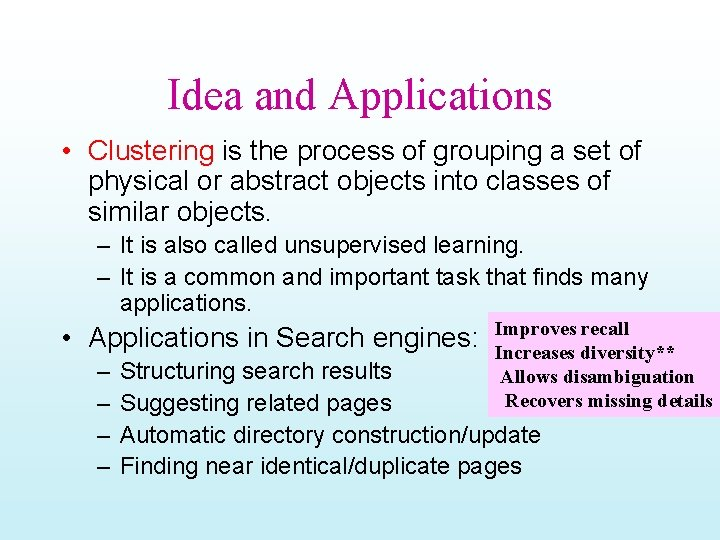Idea and Applications • Clustering is the process of grouping a set of physical or abstract objects into classes of similar objects. – It is also called unsupervised learning. – It is a common and important task that finds many applications. • Applications in Search engines: – – Improves recall Increases diversity** Allows disambiguation Recovers missing details Structuring search results Suggesting related pages Automatic directory construction/update Finding near identical/duplicate pages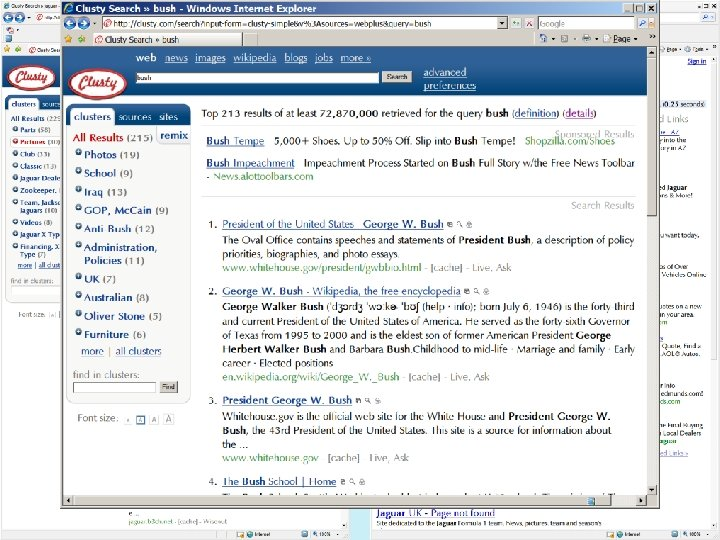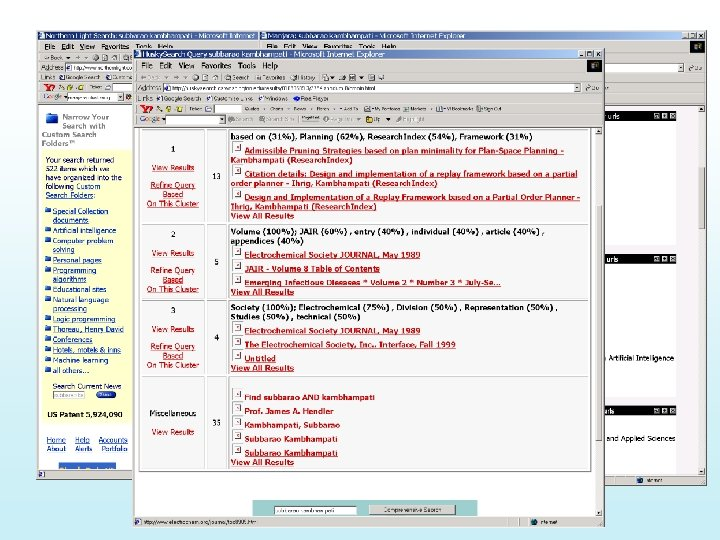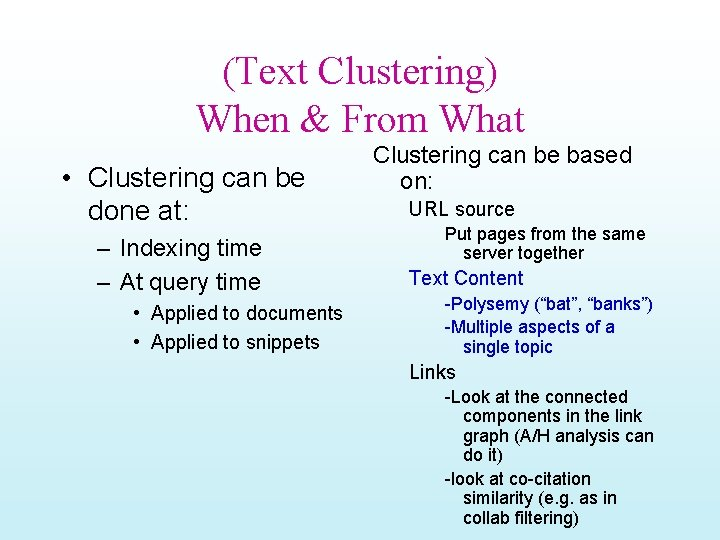(Text Clustering) When & From What • Clustering can be done at: – Indexing time – At query time • Applied to documents • Applied to snippets Clustering can be based on: URL source Put pages from the same server together Text Content -Polysemy (“bat”, “banks”) -Multiple aspects of a single topic Links -Look at the connected components in the link graph (A/H analysis can do it) -look at co-citation similarity (e. g. as in collab filtering)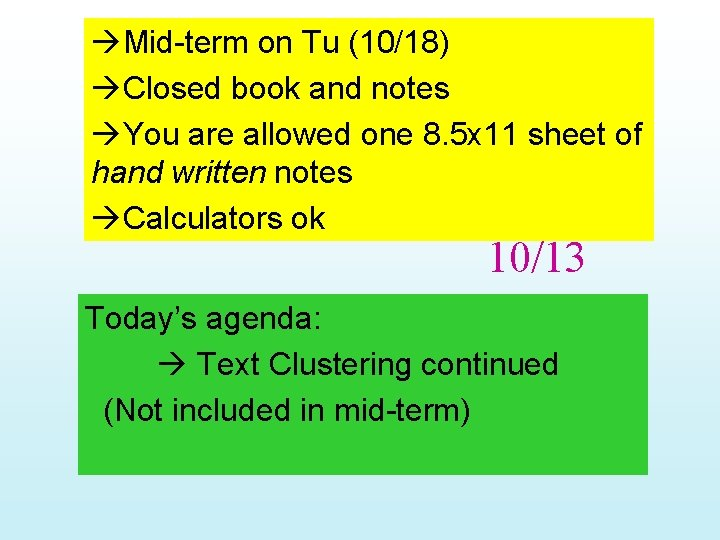Mid-term on Tu (10/18) Closed book and notes You are allowed one 8. 5 x 11 sheet of hand written notes Calculators ok 10/13 Today’s agenda: Text Clustering continued (Not included in mid-term)Clustering issues --Hard vs. Soft clusters --Distance measures cosine or Jaccard or. . --Cluster quality: Internal measures --intra-cluster tightness --inter-cluster separation External measures --How many points are put in wrong clusters. [From Mooney]Cluster Evaluation – “Clusters can be evaluated with “internal” as well as “external” measures • Internal measures are related to the inter/intra cluster distance – A good clustering is one where » (Intra-cluster distance) the sum of distances between objects in the same cluster are minimized, » (Inter-cluster distance) while the distances between different clusters are maximized » Objective to minimize: F(Intra, Inter) • External measures are related to how representative are the current clusters to “true” classes. Measured in terms of purity, entropy or F-measure – Note that in real world, you often don’t know what the true classes are. (This is why clustering is called unsupervised learning)Inter/Intra Cluster Distances Intra-cluster distance/tightness • (Sum/Min/Max/Avg) the (absolute/squared) distance between - All pairs of points in the cluster OR - “diameter”—two farthest points - Between the centroid /medoid and all points in the cluster Inter-cluster distance Sum the (squared) distance between all pairs of clusters Where distance between two clusters is defined as: - distance between their centroids/medoids - Distance between farthest pair of points (complete link) - Distance between the closest pair of points belonging to the clusters (single link) Letter grades and Single vs. Complete link Red: Single-link Black: complete-link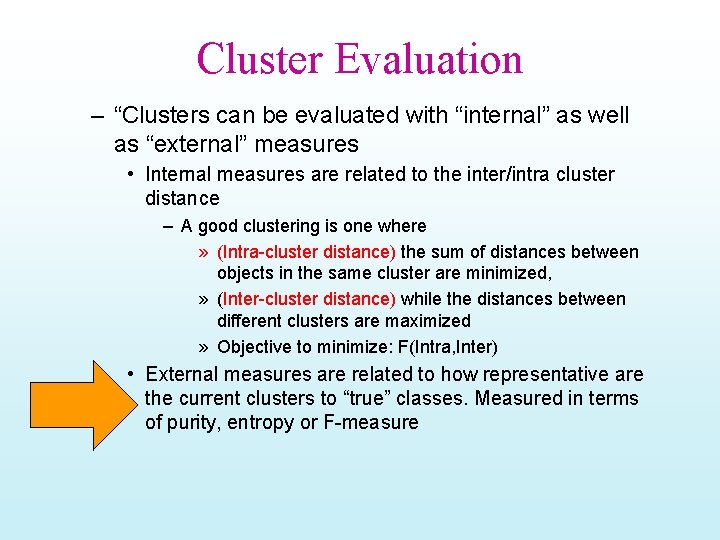Cluster Evaluation – “Clusters can be evaluated with “internal” as well as “external” measures • Internal measures are related to the inter/intra cluster distance – A good clustering is one where » (Intra-cluster distance) the sum of distances between objects in the same cluster are minimized, » (Inter-cluster distance) while the distances between different clusters are maximized » Objective to minimize: F(Intra, Inter) • External measures are related to how representative are the current clusters to “true” classes. Measured in terms of purity, entropy or F-measure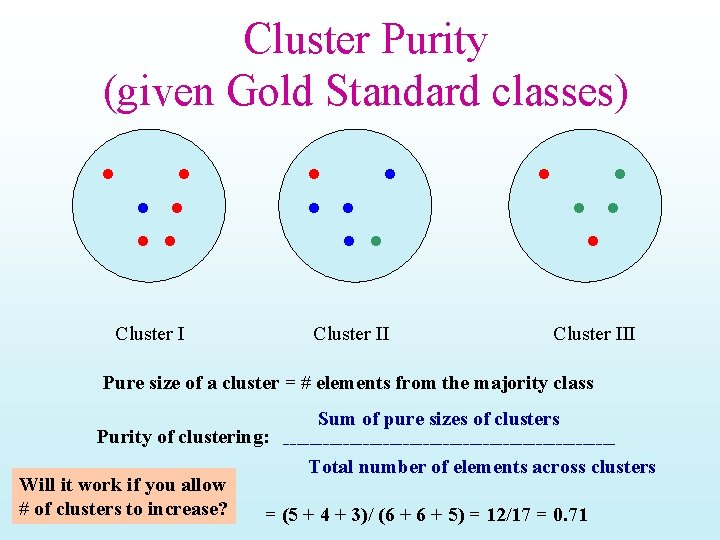Cluster Purity (given Gold Standard classes) Cluster III Pure size of a cluster = # elements from the majority class Sum of pure sizes of clusters Purity of clustering: -------------------------Total number of elements across clusters Will it work if you allow # of clusters to increase? = (5 + 4 + 3)/ (6 + 5) = 12/17 = 0. 71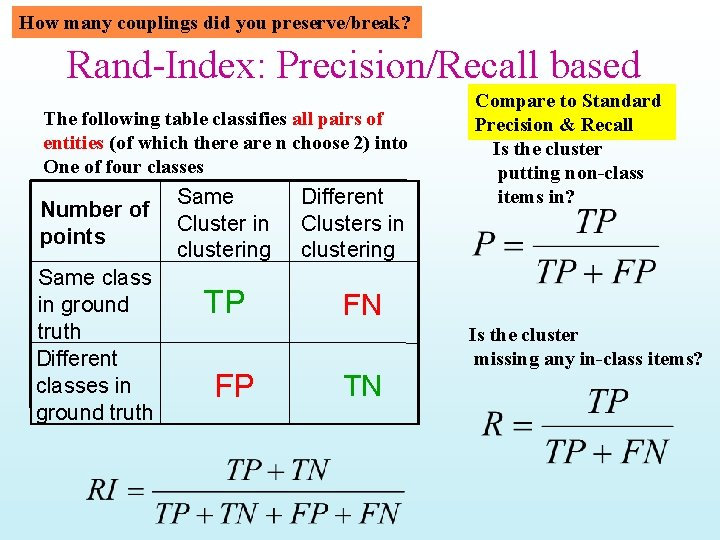How many couplings did you preserve/break? Rand-Index: Precision/Recall based The following table classifies all pairs of entities (of which there are n choose 2) into One of four classes Number of points Same class in ground truth Different classes in ground truth Same Cluster in clustering TP FP Different Clusters in clustering Compare to Standard Precision & Recall Is the cluster putting non-class items in? FN Is the cluster missing any in-class items? TN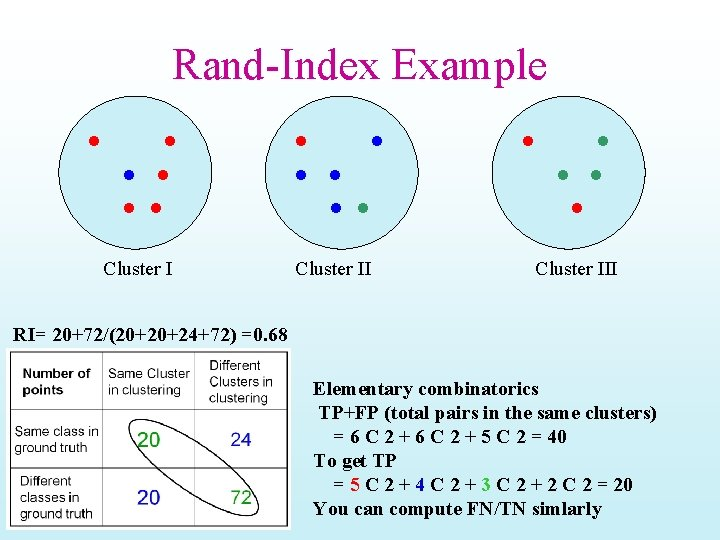Rand-Index Example Cluster III RI= 20+72/(20+20+24+72) =0. 68 Elementary combinatorics TP+FP (total pairs in the same clusters) = 6 C 2 + 5 C 2 = 40 To get TP = 5 C 2 + 4 C 2 + 3 C 2 + 2 C 2 = 20 You can compute FN/TN simlarly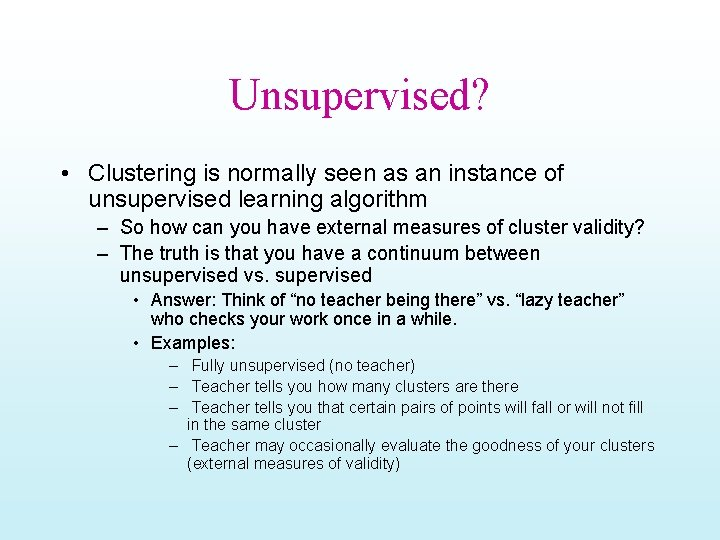Unsupervised? • Clustering is normally seen as an instance of unsupervised learning algorithm – So how can you have external measures of cluster validity? – The truth is that you have a continuum between unsupervised vs. supervised • Answer: Think of “no teacher being there” vs. “lazy teacher” who checks your work once in a while. • Examples: – Fully unsupervised (no teacher) – Teacher tells you how many clusters are there – Teacher tells you that certain pairs of points will fall or will not fill in the same cluster – Teacher may occasionally evaluate the goodness of your clusters (external measures of validity)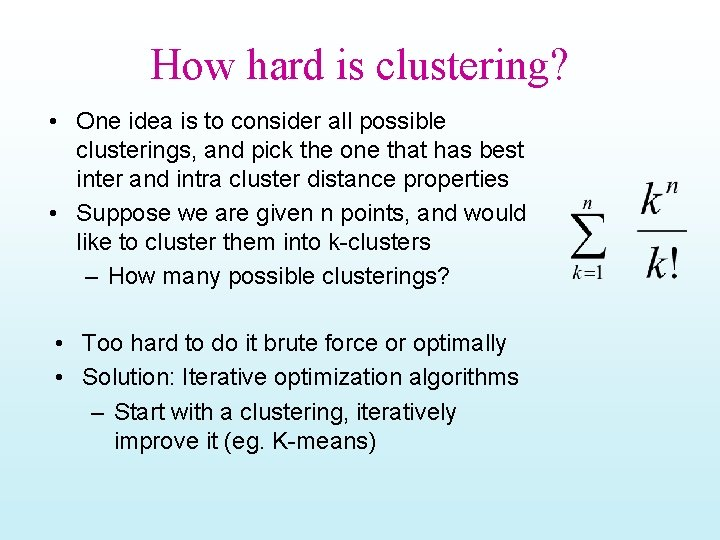How hard is clustering? • One idea is to consider all possible clusterings, and pick the one that has best inter and intra cluster distance properties • Suppose we are given n points, and would like to cluster them into k-clusters – How many possible clusterings? • Too hard to do it brute force or optimally • Solution: Iterative optimization algorithms – Start with a clustering, iteratively improve it (eg. K-means)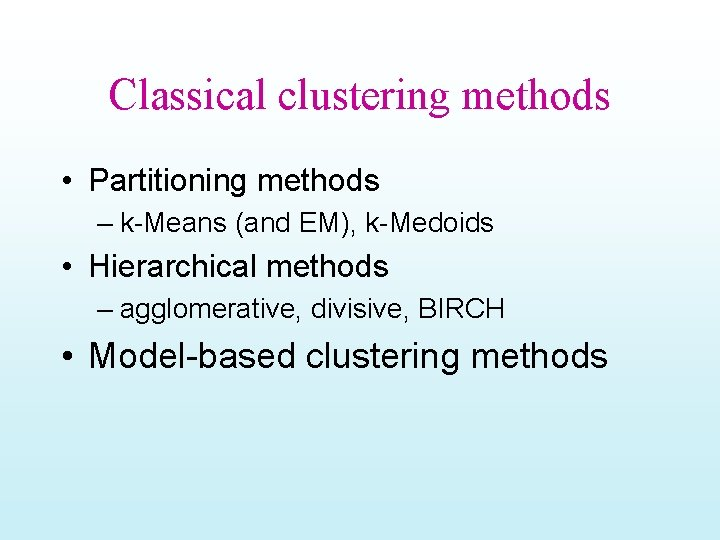Classical clustering methods • Partitioning methods – k-Means (and EM), k-Medoids • Hierarchical methods – agglomerative, divisive, BIRCH • Model-based clustering methods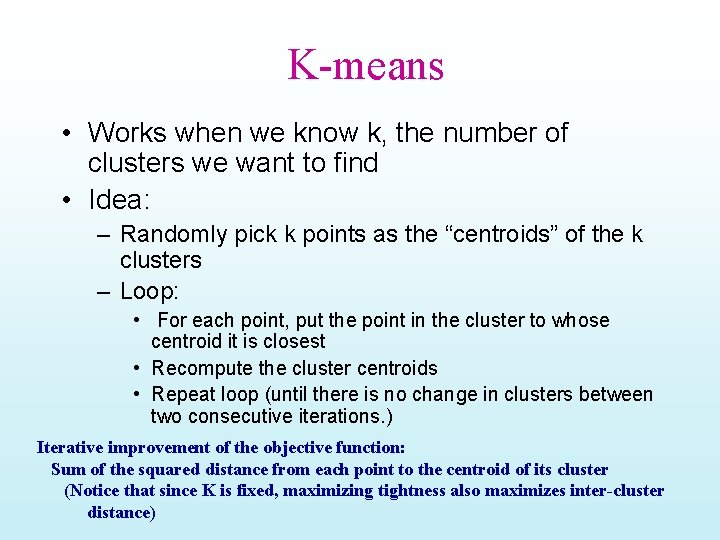K-means • Works when we know k, the number of clusters we want to find • Idea: – Randomly pick k points as the “centroids” of the k clusters – Loop: • For each point, put the point in the cluster to whose centroid it is closest • Recompute the cluster centroids • Repeat loop (until there is no change in clusters between two consecutive iterations. ) Iterative improvement of the objective function: Sum of the squared distance from each point to the centroid of its cluster (Notice that since K is fixed, maximizing tightness also maximizes inter-cluster distance)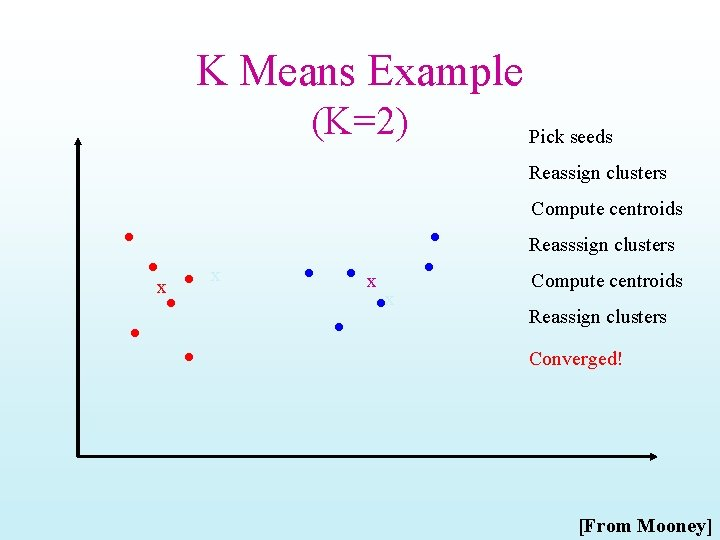K Means Example (K=2) Pick seeds Reassign clusters Compute centroids Reasssign clusters x x Compute centroids Reassign clusters Converged! [From Mooney]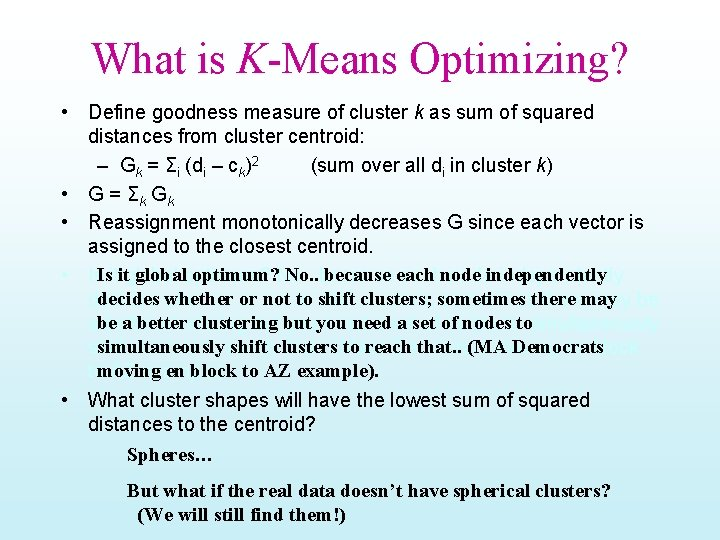What is K-Means Optimizing? • Define goodness measure of cluster k as sum of squared distances from cluster centroid: – Gk = Σi (di – ck)2 (sum over all di in cluster k) • G = Σk G k • Reassignment monotonically decreases G since each vector is assigned to the closest centroid. globaloptimum? No. . because each • Is. Isititglobal eachnodeindependently decides whether or not to shift clusters; decides clusters; sometimestheremay be a better clustering need a set nodestotosimultaneously abe better clustering but youyou need a set of of nodes simultaneously shift clusters to reach that. . (MA Democrats shift clusters to reach that. . (Mass Democrats moving en block to AZ example). tomoving AZ example). • What cluster shapes will have the lowest sum of squared distances to the centroid? Spheres… But what if the real data doesn’t have spherical clusters? (We will still find them!)K-means Example • For simplicity, 1 -dimension objects and k=2. – Numerical difference is used as the distance • Objects: 1, 2, • K-means: 5, 6, 7 – Randomly select 5 and 6 as centroids; – => Two clusters {1, 2, 5} and {6, 7}; mean. C 1=8/3, mean. C 2=6. 5 – => {1, 2}, {5, 6, 7}; mean. C 1=1. 5, mean. C 2=6 – => no change. – Aggregate dissimilarity • (sum of squares of distanceeach point of each cluster from its cluster center--(intra-cluster distance) – = 0. 52+ 12+ 02+12 = 2. 5 |1 -1. 5|2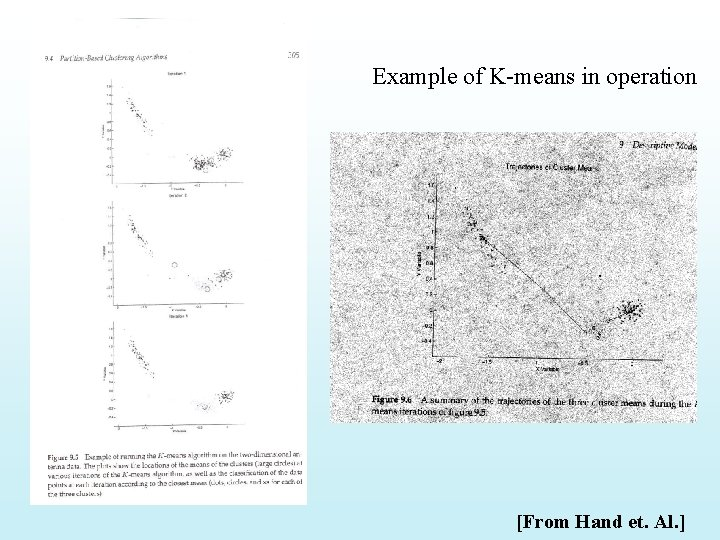Example of K-means in operation [From Hand et. Al. ]10/20Problems with. Why. K-means not the • Need to know k in advance – Could try out several k? minimum value? • Cluster tightness increases with increasing K. – Look for a kink in the tightness vs. K curve • Tends to go to local minima that are sensitive to the starting centroids – Try out multiple starting points • Disjoint and exhaustive – Doesn’t have a notion of “outliers” • Outlier problem can be handled by K -medoid or neighborhood-based algorithms • Assumes clusters are spherical in vector space – Sensitive to coordinate changes, weighting etc.Looking for knees in the sum of intra-cluster dissimilarity The problem is that Comparing G values across different clustering sizes (k) is apple/organge comparison Once k is allowed to change we need to also consider inter-cluster distance that is not captured by GPenalize lots of clusters • For each cluster, we have a Cost C. • Thus for a clustering with K clusters, the Total Cost is KC. • Define the Value of a clustering to be = Total Benefit - Total Cost. • Find the clustering of highest value, over all choices of K. – Total benefit increases with increasing K. But can stop when it doesn’t increase by “much”. The Cost term enforces this.Problems with K-means • Need to know k in advance – Could try out several k? • Cluster tightness increases with increasing K. Example showing sensitivity to seeds – Look for a kink in the tightness vs. K curve • Tends to go to local minima that are sensitive to the starting centroids – Try out multiple starting points • Disjoint and exhaustive – Doesn’t have a notion of “outliers” • Outlier problem can be handled by K -medoid or neighborhood-based algorithms • Assumes clusters are spherical in vector space – Sensitive to coordinate changes, weighting etc. In the above, if you start with B and E as centroids you converge to {A, B, C} and {D, E, F} If you start with D and F you converge to {A, B, D, E} {C, F}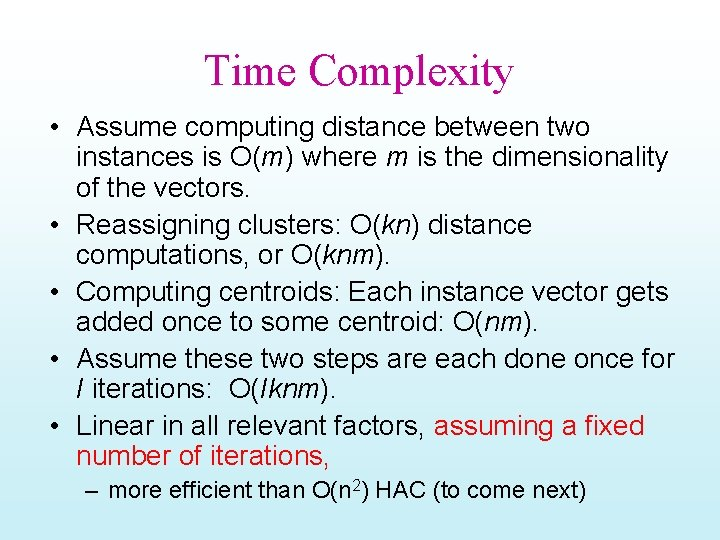Time Complexity • Assume computing distance between two instances is O(m) where m is the dimensionality of the vectors. • Reassigning clusters: O(kn) distance computations, or O(knm). • Computing centroids: Each instance vector gets added once to some centroid: O(nm). • Assume these two steps are each done once for I iterations: O(Iknm). • Linear in all relevant factors, assuming a fixed number of iterations, – more efficient than O(n 2) HAC (to come next)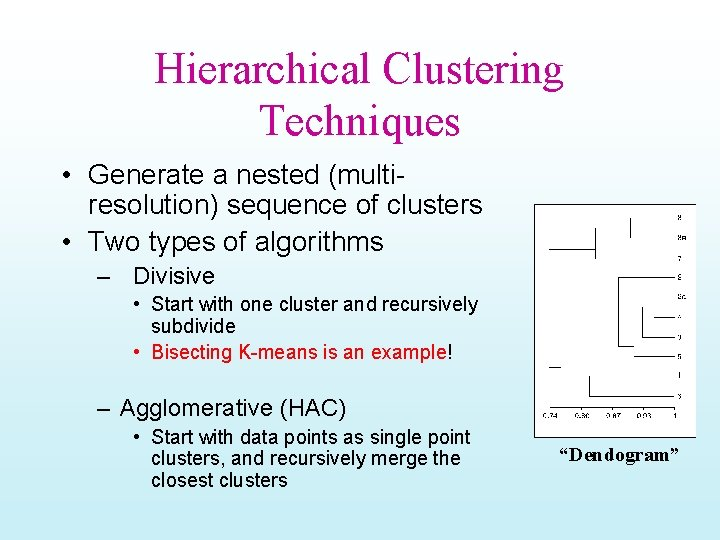Hierarchical Clustering Techniques • Generate a nested (multiresolution) sequence of clusters • Two types of algorithms – Divisive • Start with one cluster and recursively subdivide • Bisecting K-means is an example! – Agglomerative (HAC) • Start with data points as single point clusters, and recursively merge the closest clusters “Dendogram”eth m d i r yb 1 d o Bisecting K-means H • For I=1 to k-1 do{ Can pick the largest Cluster or the cluster With lowest average similarity – Pick a leaf cluster C to split – For J=1 to ITER do{ • Use K-means to split C into two sub-clusters, C 1 and C 2 • Choose the best of the above splits and make it permanent} } Divisive hierarchical clustering method uses K-means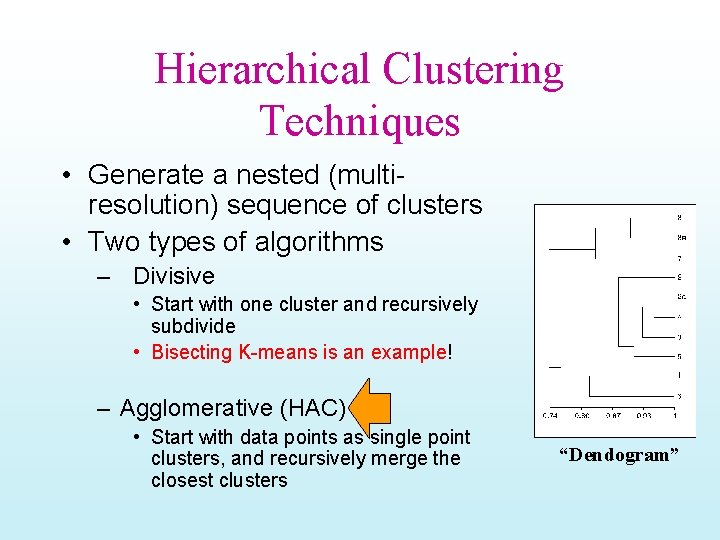Hierarchical Clustering Techniques • Generate a nested (multiresolution) sequence of clusters • Two types of algorithms – Divisive • Start with one cluster and recursively subdivide • Bisecting K-means is an example! – Agglomerative (HAC) • Start with data points as single point clusters, and recursively merge the closest clusters “Dendogram”Hierarchical Agglomerative Clustering Example • {Put every point in a cluster by itself. For I=1 to N-1 do{ let C 1 and C 2 be the most mergeable pair of clusters (defined as the two closest clusters) Create C 1, 2 as parent of C 1 and C 2} • Example: For simplicity, we still use 1 -dimensional objects. – Numerical difference is used as the distance • Objects: 1, 2, 5, 6, 7 • agglomerative clustering: – – find two closest objects and merge; => {1, 2}, so we have now {1. 5, 5, 6, 7}; => {1, 2}, {5, 6}, so {1. 5, 5. 5, 7}; => {1, 2}, {{5, 6}, 7}. 1 2 5 • Qn: What should be the distance between two clusters (or a cluster and a point) – Single-link (closest two points); multi-link (farthest two points)… 6 7Single Link ExampleComplete Link ExampleImpact of cluster distance measures “Single-Link” (inter-cluster distance= distance between closest pair of points) [From Mooney] “Complete-Link” (inter-cluster distance= distance between farthest pair of points)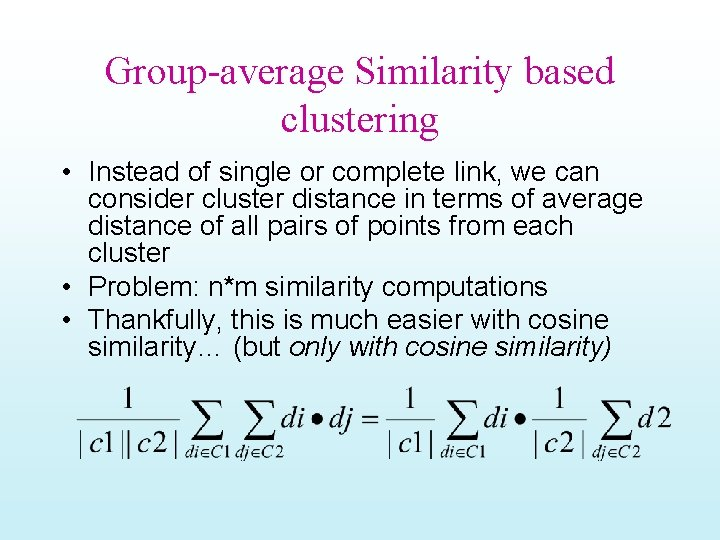Group-average Similarity based clustering • Instead of single or complete link, we can consider cluster distance in terms of average distance of all pairs of points from each cluster • Problem: n*m similarity computations • Thankfully, this is much easier with cosine similarity… (but only with cosine similarity)Properties of HAC • Creates a complete binary tree (“Dendogram”) of clusters • Various ways to determine mergeability – “Single-link”—distance between closest neighbors – “Complete-link”—distance between farthest neighbors – “Group-average”—average distance between all pairs of neighbors – “Centroid distance”—distance between centroids is the most common measure • Deterministic (modulo tie-breaking) • Runs in O(N 2) time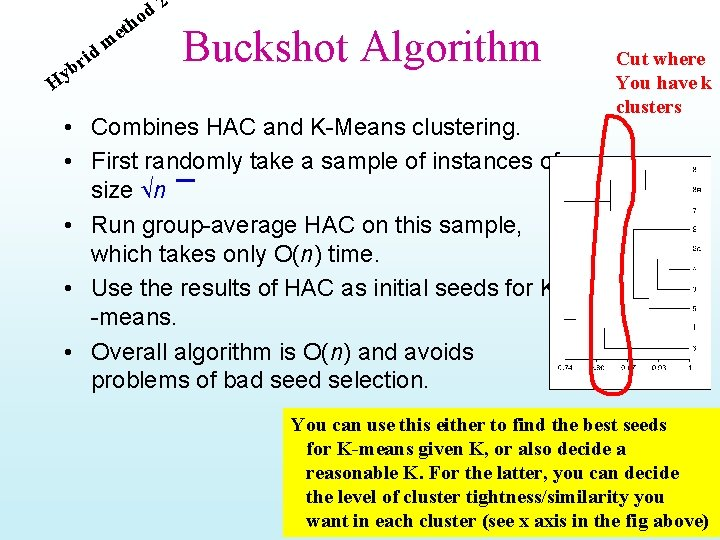eth H m d i r yb 2 d o Buckshot Algorithm • Combines HAC and K-Means clustering. • First randomly take a sample of instances of size n • Run group-average HAC on this sample, which takes only O(n) time. • Use the results of HAC as initial seeds for K -means. • Overall algorithm is O(n) and avoids problems of bad seed selection. Cut where You have k clusters You can use this either to find the best seeds for K-means given K, or also decide a reasonable K. For the latter, you can decide the level of cluster tightness/similarity you want in each cluster (see x axis in the fig above)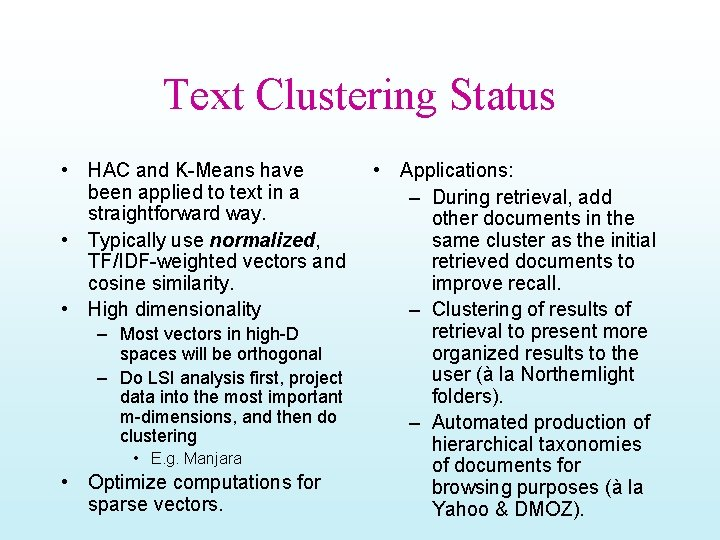Text Clustering Status • HAC and K-Means have been applied to text in a straightforward way. • Typically use normalized, TF/IDF-weighted vectors and cosine similarity. • High dimensionality – Most vectors in high-D spaces will be orthogonal – Do LSI analysis first, project data into the most important m-dimensions, and then do clustering • E. g. Manjara • Optimize computations for sparse vectors. • Applications: – During retrieval, add other documents in the same cluster as the initial retrieved documents to improve recall. – Clustering of results of retrieval to present more organized results to the user (à la Northernlight folders). – Automated production of hierarchical taxonomies of documents for browsing purposes (à la Yahoo & DMOZ).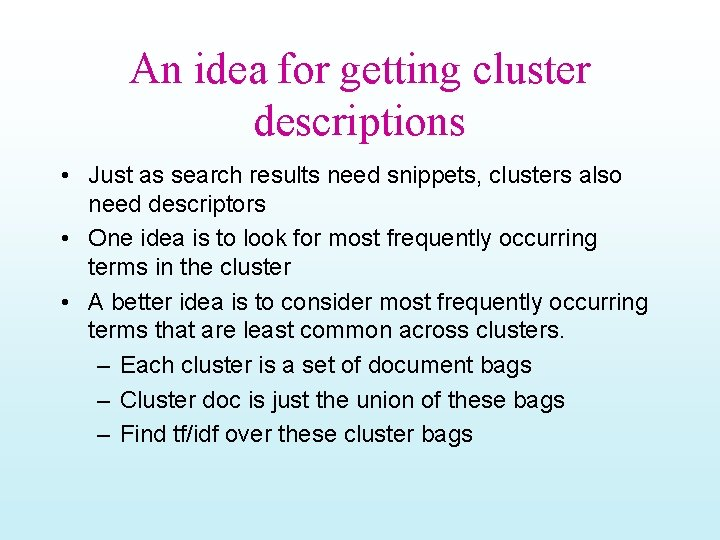An idea for getting cluster descriptions • Just as search results need snippets, clusters also need descriptors • One idea is to look for most frequently occurring terms in the cluster • A better idea is to consider most frequently occurring terms that are least common across clusters. – Each cluster is a set of document bags – Cluster doc is just the union of these bags – Find tf/idf over these cluster bagsDidn’t cover after this. .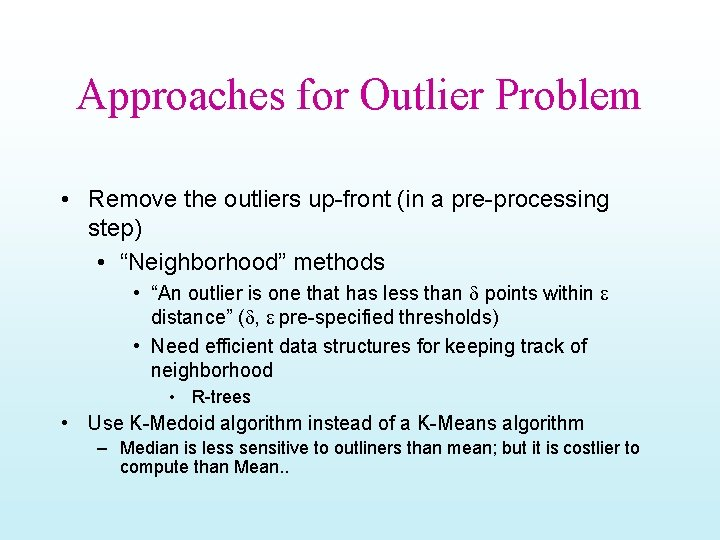Approaches for Outlier Problem • Remove the outliers up-front (in a pre-processing step) • “Neighborhood” methods • “An outlier is one that has less than d points within e distance” (d, e pre-specified thresholds) • Need efficient data structures for keeping track of neighborhood • R-trees • Use K-Medoid algorithm instead of a K-Means algorithm – Median is less sensitive to outliners than mean; but it is costlier to compute than Mean. .Variations on K-means (contd) • Outlier problem – Use K-Medoids • Costly! • Non-hard clusters – Use soft K-means {basically, EM} • Let the membership of each data point in a cluster be proportional to its distance from that cluster center • Membership weight of elt e in cluster C is set to – Exp(-b dist(e; center(C)) » Normalize the weight vector – Normal K-means takes the max of weights and assigns it to that cluster » The cluster center re-computation step is based on the membership – We can instead let the cluster center computation be based on the all points, weighted by their membership weightAdded after class discussion; optional K-Means & Expectation Maximization • • A “model-based” clustering scenario The data points were generated from k Gaussians N(mi, vi) with mean mi and variance vi In this case, clearly the right clustering involves estimating the mi and vi from the data points We can use the following iterative idea: – Initialize: guess estimates of mi and vi for all k gaussians – Loop It is easy to see that K-means is a “hard-assignment” Form of EM procedure For recovering the Model parameters • • • (E step): Compute the probability Pij that ith point is generated by jth cluster (which is simply the value of normal distribution N(mj, vj) at the point di ). {Note that after this step, each point will have k probabilities associated with its membership in each of the k clusters) • (M step): Revise the estimates of the mean and variance of each of the clusters taking into account the expected membership of each of the points in each of the clusters Repeat It can be proven that the procedure above converges to the true means and variances of the original k Gaussians (Thus recovering the parameters of the generative model) The procedure is a special case of a general schema for probabilistic algorithm schema called “Expectation Maximization”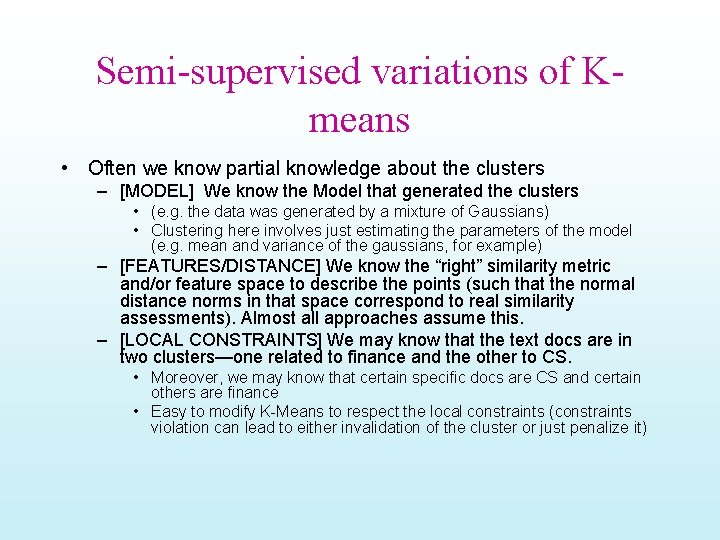Semi-supervised variations of Kmeans • Often we know partial knowledge about the clusters – [MODEL] We know the Model that generated the clusters • (e. g. the data was generated by a mixture of Gaussians) • Clustering here involves just estimating the parameters of the model (e. g. mean and variance of the gaussians, for example) – [FEATURES/DISTANCE] We know the “right” similarity metric and/or feature space to describe the points (such that the normal distance norms in that space correspond to real similarity assessments). Almost all approaches assume this. – [LOCAL CONSTRAINTS] We may know that the text docs are in two clusters—one related to finance and the other to CS. • Moreover, we may know that certain specific docs are CS and certain others are finance • Easy to modify K-Means to respect the local constraints (constraints violation can lead to either invalidation of the cluster or just penalize it)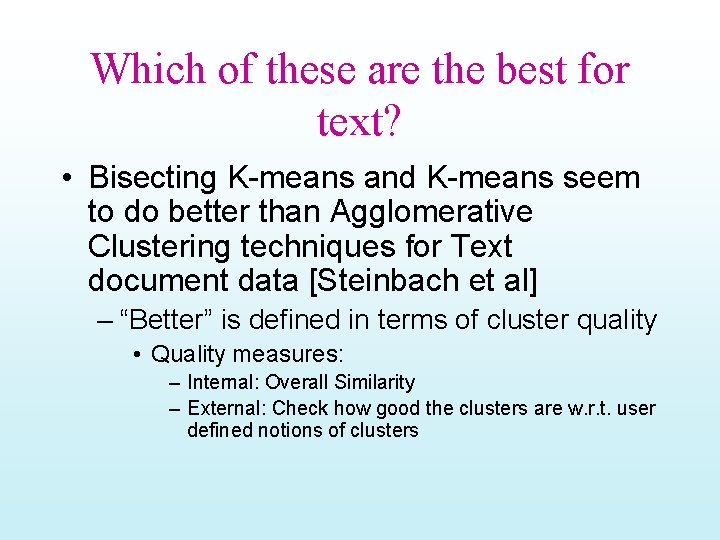Which of these are the best for text? • Bisecting K-means and K-means seem to do better than Agglomerative Clustering techniques for Text document data [Steinbach et al] – “Better” is defined in terms of cluster quality • Quality measures: – Internal: Overall Similarity – External: Check how good the clusters are w. r. t. user defined notions of clusters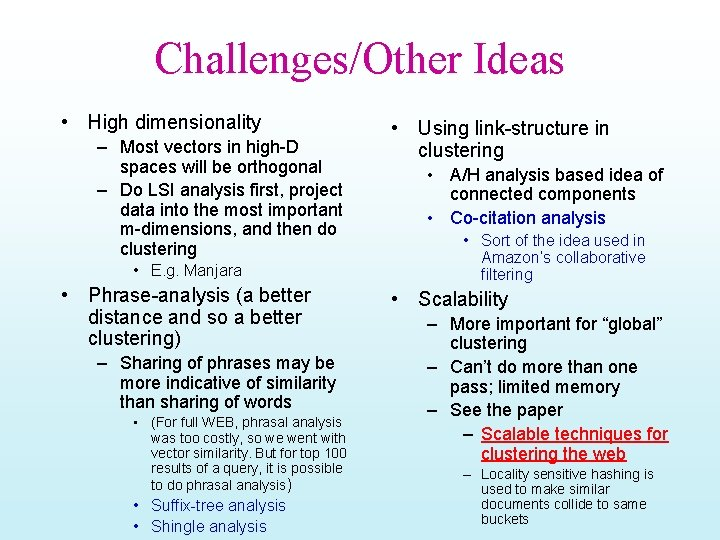Challenges/Other Ideas • High dimensionality – Most vectors in high-D spaces will be orthogonal – Do LSI analysis first, project data into the most important m-dimensions, and then do clustering • E. g. Manjara • Phrase-analysis (a better distance and so a better clustering) – Sharing of phrases may be more indicative of similarity than sharing of words • (For full WEB, phrasal analysis was too costly, so we went with vector similarity. But for top 100 results of a query, it is possible to do phrasal analysis) • Suffix-tree analysis • Shingle analysis • Using link-structure in clustering • A/H analysis based idea of connected components • Co-citation analysis • Sort of the idea used in Amazon’s collaborative filtering • Scalability – More important for “global” clustering – Can’t do more than one pass; limited memory – See the paper – Scalable techniques for clustering the web – Locality sensitive hashing is used to make similar documents collide to same buckets